A rack and pinion drive features a circular gear (also known as the pinion) that engages a linear gear (the rack) to convert revolving motion into linear motion. Although commonly dismissed by most engineers as an obsolete technology, rack and pinion drives offer a combination of high thrust and high speed at relatively low cost. But this performance-price advantage will only be felt if the rack and pinion drive is correctly sized.

## Key specifications for rack and pinion drives

The sizing of rack and pinion drive is typically based on three factors:

1. Tangential force on the rack

2. Torque

3. The rotational speed of the pinion

### Tangential force

Tangential force, also known as feed force, is the force required to deliver the linear movement profile. The magnitude of this force usually varies depending on the application requirements, which could either be horizontal or vertical.

Considering horizontal applications, where the load moves opposite the direction of the guide system, the rack experiences two types of forces:

1. A force due to the friction of the guide rails

2. A force resulting from the acceleration of the mass

Tangential force in such applications can be calculated using: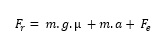Tangential force equation for horizontal applications.

Where:

Fr = tangential force on the rack (N)

m = mass of components being moved (kg)

g = gravitational constant (9.81 m/s2)

µ = coefficient of friction of the guide

a = acceleration that the mass will experience (m/s2)

Fe = additional external forces resulting from the movement of the drive (N).

In vertical applications, the load moves in the direction of the guide system. As such, it is not affected by the friction force from the guide rails. It can be calculated using: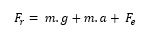Tangential force equation for vertical applications.

### Torque

Torque is the rotational equivalent of a linear force. In simple terms, it is the pinion’s rotational force. It is given as: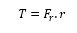Rack and pinion torque equation 1.

Where:

T = torque on pinion (Nm)

Fr = Tangential force on the rack

r = radius of the pinion (m)

Torque can also be calculated using: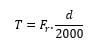Rack and pinion torque equation 2.

Where:

d = diameter of the pinion (in millimeters)

## Pinion rotational speed

The rotational speed of the pinion, expressed in revolutions per minute (rpm), is the number of turns the pinion can make about its axis in one minute. The maximum rotational speed of the pinion can be calculated using: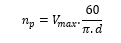Pinion maximum RPM equation 1.

Where:

Vmax = maximum linear speed of the application (m/s)

d = diameter of the pinion (m)

np = maximum rotational speed (rpm)

Since the dimensions of the pinion are often expressed in millimeters, the maximum rotational speed can also be calculated using the following equations.: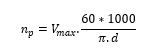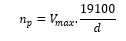Pinion maximum RPM equation 2.

Where:

d = diameter of the pinion (millimeters)

Before taking the plunge with these formulas, engineers must consider using safety factors when determining the force on the rack. Manufacturers of rack and pinion drive typically recommend a safety factor ranging between 2 and 3 for both horizontal and vertical applications.

## Further considerations

There’s much more to rack and pinion drive design than mechanical sizing. Engineers still have to determine the ideal gear quality, strength and hardness for their applications.

Here are a few helpful tips for rack and pinion gear design:

1. Choose an engineering material with sufficient strength to prevent the gear teeth from failing under static loading.

2. Choose an engineering material with good wear characteristics to prolong the service life of the gear.

3. Note that larger pinions provide more backlash, while smaller pinions are ideal for transmitting lower torques.

Product designers should consider cross-checking several standards, including ISO 1328-1 and AGMA 2015-2, for more information.

Reach out to rack and pinion suppliers or manufacturers for application specific questions or solutions.